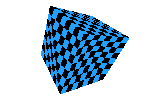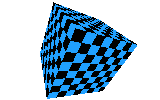Perspective Texturemapping
using linear interpolation of 1/Z, U/Z and V/Z

An article written for 3DReview in 1997 by
Mikael Kalms

Introduction

Linear texturemappers are in common use in the demos of today. Most games have however progressed to perspective corrected texturemapping, and soon most demos probably will follow. 3D graphics cards usually perform perspective correction for almost no extra cost (speaking in terms of speed), but there are still not enough many machines to let any program require 3D graphics hardware -- software rendering fallback code is still necessary.

This article will describe a method of performing perspective corrected texture mapping which suits the Pentium processor (and most of its clones) very well. The most notable exception to this are Cyrix' clones which have a relatively weak FPU and therefore suffer from performance degradations in every part of the texturemapper.

What does it look like?

Here are some screenshots of triangulated cubes drawn with a blue/black chequered texture. Click on the pictures to see the bigger versions.Linear Perspective corrected at every pixel Perspective corrected at every 16th pixel horizontally [Download Executable Example] [Download Executable Example] [Download Executable Example]

NB: Example executables require DOS4GW (not included) Run executables with any parameter to have chequered pattern instead of default texture.

Let's Get to It

It is assumed that the readers are familiar with linear texturemappers and have some maths knowledge about derivatives and linear interpolation. A fair bit of C knowledge will also be helpful for the sources.

Several approaches for performing perspective corrected tmapping is available. The Pentium relies heavily on its internal caches; therefore drawing the polygon as a set of horizontal lines is strongly preferred. (This rules out any methods such as "drawing lines of constant Z".) The Pentium also has quite a strong FPU (with the exception of the Cyrix clones, and possibly some others as well). Floating point math can therefore be used to a small degree.

The method which now will be described relies on the fact that 1/z, u/z and v/z are linear in screen space, and thus can be interpolated linearly without any errors (except for accuracy errors, of course).

Proving that 1/z, u/z and v/z are linear in screen space

This section is not required to be understood - it is only provided for those who wish to have mathematical proof of the theorem that the algorithm is based on.

"a is a linear function of xyz" means that linear (constant-velocity) motion along the xyz axes will translate into linear motion along a's coordinate axis. If one imagines xyz visually, one could also say that "a is linear in xyz space", which means just the same. The above relationship is expressed like this with formulas:

a = k1x + k2y + k3z + k4

XY are perspective projected, 2d screen coordinates.
xyz are coordinates in 3d space of points on the polygon.
uv are texture coordinates in the texture that is going to be mapped onto the polygon.
abcd and efgh are coefficients for the definition of uv. They are constant for the whole polygon.
ABCD are the coefficients of the plane which the poly lies in. They are constant for the whole polygon.

The relationship between XY and xyz is as follows:

X = x/z
Y = y/z

...which is the usual perspective projection formula.
Solving those for x and y gives:

x = Xz
y = Yz

These equations will come in handy later on.

We only want xyz combinations that are on the plane which the polygon lies on. Therefore all the xyz combinations we are interested in are solutions to the following equation:

Ax + By + Cz = D

uv need to be defined in relation to xyz.
The equations below describe uv as linear functions of xyz:

u = ax + by + cz + d
v = ex + fy + gz + h

1) Proving that 1/z is linear in XY space

Beginning with the plane equation:

Ax + By + Cz = D

Substituting xy with the formulas from the perspective projection:

AXz + BYz + Cz = D

Solving for 1/z (dividing by (AX + BY + C)) yields:

1/z = (A/D)X + (B/D)Y + (C/D)

Here it is clearly visible that 1/z is a linear function of XY (1/z = k1X + k2Y + k3). 1/z is thus linear in screen space.

2) Proving that u/z, v/z are linear in screen space

Beginning with the uv formulas:

u = ax + by + cz + d
v = ex + fy + gz + h

Substituting xy with the formulas from the perspective projection:

u = aXz + bYz + cz + d
v = eXz + fYz + gz + h

Dividing by z:

u/z = (aX + bY + c) + d/z
v/z = (eX + fY + g) + h/z

Substituting the 1/z with the formula from the previous calculation:

u/z = aX + bY + c + d((A/D)X + (B/D)Y + (C/D))
v/z = eX + fY + g + h((A/D)X + (B/D)Y + (C/D))

Cleaning up the equation a little gives these final formulas:

u/z = (a + d(A/D))X + (b + d(B/D))Y + (c + d(C/D))
v/z = (e + h(A/D))X + (f + h(B/D))Y + (g + h(C/D))

Here one can also see that both u/z and v/z are on the form k1X + k2Y + k3.
u/z and v/z are thus also linear in screen space.

Now then, let's do the tmapper

This is the easy part. :)
Interpolating 1/z, u/z and v/z over the polygon and then calculating uv from those values will give correct uv's, so that's what should be done.

At each vertex in the polygon, compute 1/z, u/z and v/z. Compute their slopes along the polygon's edges, and their horizontal increases. (Since these values are truly linear on screen, the horizontal increase values are constant for all planar polygons, not only triangles!) Interpolate the three factors along the edges, then horizontally, and at each pixel perform these computations to get uv:

z = 1 / (1/z)
u = (u/z) * z
v = (v/z) * z

Then copy the matching pixel from the texture to the screen.

This method has a very low setup cost, but the per-pixel cost is relatively high compared to other perspective correction methods. In order to remedy that, a partially approximative method is possible, as shown in the next paragraph.

Scanline subdivision

Subdividing each horizontal line in the polygon into spans of length N pixels each has proven to be a effective approximation method. N should preferably be a power of 2; typical values for N are 8 or 16.

The subdivision is done on-the-fly in the code part that draws the horizontal line:

1/z, u/z and v/z are stepped N pixels at a time instead of 1. (Pre-multiply the horizontal increases by N when calculating them.) Calculate uv at the current position (x) and at position (x + N), and draw the pixels from (x) to (x + N - 1) using normal linear uv interpolation. The horizontal uv-increases are computed by doing (u2 - u1) >> log2(N) and (v2 - v1) >> log2(N).

This will remove much of the per-pixel cost, yet retain a very high level of quality.

By hand-writing the horizontal line drawer in assembly language there is yet another feature to take advantage of: CPU/FPU parallelism. [This holds true mostly for the Pentium; the 486's FPU is way slower than the 486 CPU] The reciprocal calculation (z = 1 / (1/z)) is what takes the most time; by interleaving the uv-calculations for position (x + 2 * N) with the code that draws the span from pixel (x) to pixel (x + N - 1), much of the overhead of the uv calculation will disappear.

The last span in each horizontal line needs special-case code as it is shorter than N pixels. uv should be calced at the exact end of the span, not at the next (x + N) position.

Sources

Here are example sources for the texture mapping approaches mentioned above. They are not optimized, they are only provided as detailed examples of how the algorithms work. The sources also implement subpixeling and subtexeling; without those features perspective correction reallty isn't much to yell about. :)

[TPOLY.CPP]
Example source of linear texturemapper

[PTPOLY1.CPP]
Example source of "perfect" perspective corrected texturemapper

[PTPOLY2.CPP]
Example source of scanline subdivision texturemapper

[DCDX.TXT]
A note on the dc/dx calculations in the example sources